How to secure a marketplace where you could buy and sell digital goods when the blockchain is public ?

While scrolling and reading some articles on Google News, I told myself what a great tool is that all is gathered in one place for the users. No need to crawl the internet, to find information or news! But as always centralisation has also its downside. This platform aims to empower the so much Google against the publishers, that it has become an inevitable source of tension with the revenue generated by the ads. What if the blockchain could help to promote, share, and distribute public and/or private content? This idea led me to propose a concept of a decentralized marketplace where authors could publish and sell their content.

# Marketplace concept and design blueprint

## Marketplace concept

Nowadays, when you want to sell a good you need to register on a centralized platform (for example Facebook Marketplace), to be able to share your ad with potential customers. The concept is to replace the centralized platform with a decentralized one so that nobody gains money and power from it except the author. The blockchain is the perfect technology for that purpose by its very means to be decentralized, however, the content of it is public. By public, I want to say that everybody can access the content of the blockchain and read its information. It can be problematic if your good is private, like your e-book. If you just publish it on a distributed web media protocol (like IPFS for example), everybody will be able to read it and therefore nobody would buy it. The solution would be to encrypt the e-book so that even if published on the blockchain nobody could read it without the private key to decrypt it.

This concept can be applied to any numeric content, video, music, or text, … but in the end, you still need to exchange (buy/sell) the private keys to be able to access the content.

A private key is very like a password, and sharing a password on the blockchain ends to converge to the same issue, everybody could access it. However, there is a solution! Let’s discover it in the next section.

## Private key exchange

First, to explain how a private key exchange can be done in a private manner on the (public) blockchain, we need to clearly understand a few concepts with asymmetric cryptography.

To perform the different operations in asymmetric cryptography you will use two keys. One private and one public. Let’s see the example in Figure 1. , if Bob wants to receive a message, Bob will provide his public key to let Max encrypt the message and use Bob's private key to decrypt the message.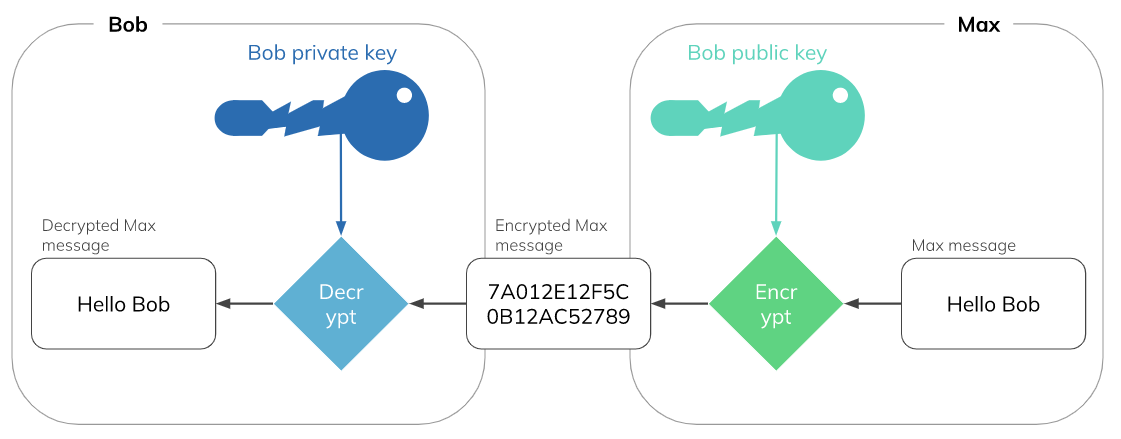Figure 1: Message encryption with public and private key pair.

The example in Figure 2. shows how to verify the message sender. To let Bob knows that it’s effectively Max that sends the message Max can sign it with his private key. Bob can use Max’s public key to verify the signature of the message and acknowledge that Max is the sender.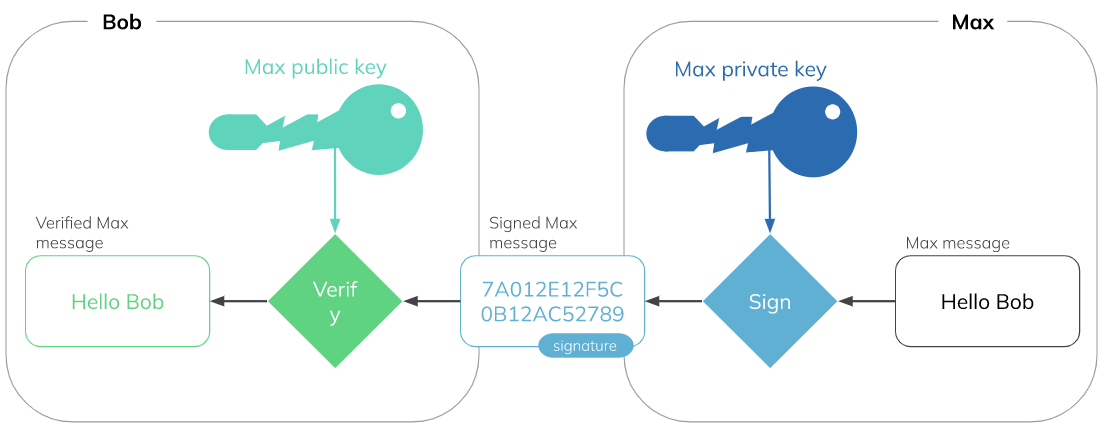Figure 2: Message signature validation with public and private key pair.

Now, that we explained the basic concepts of asymmetric cryptography, let’s define a strategy to exchange a private key in a private manner on the blockchain, like if Eva would like to buy an article. For that purpose, we would have to sell the article's private key to decrypt/read it. Therefore the system has to send the article's private key to Eva, but as on the blockchain everything is public we have to encrypt the article's private key with Eva's public key so that only Eva can access the key.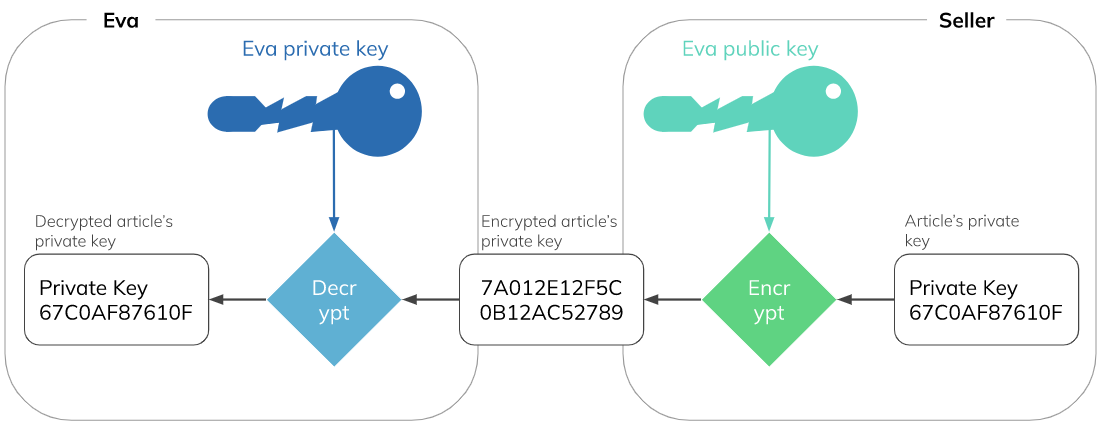Figure 3: The seller encrypts and sends the private key that Eva bought.

Eva can easily assert that the article’s private key sold is the good one, by using the article’s public key. But what if the seller tried to scam Eva by selling a fraudulent key? Before releasing the “money” to the seller, Eva has to validate or invalidate the transaction within a time limit. If the key is fraudulent, she can invalidate it by sending the wrong private key to the blockchain. Then the blockchain will compare the wrong article’s key, with the article’s public key, to assert that the key is effectively wrong and then will encrypt the wrong article’s private key to compare it with the previously sent encrypted article’s private key by the sender. If they are not equal it means that the Seller sent the wrong key and is a scam.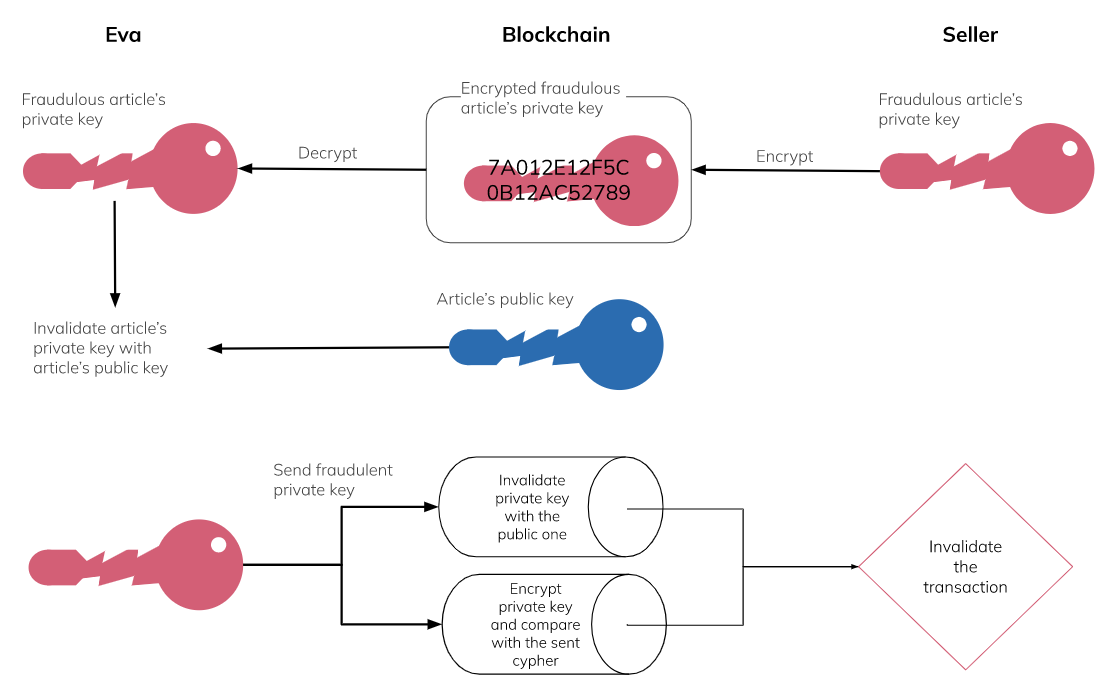Figure 4: Private key verification process. How can the buyer dismiss the transaction and the blockchain verify the scam ?

To be able to complete this verification schema, we need to implement a smart contract two behaviour: first the private key validation from a public key, and secondly the encryption with a public key.

# Implementation

## Private key validation from a public key

To validate that the private key comes from the same owner/author, we can use the public key. The public key is generated from the private key. Therefore we can use the private key to generate the public and compare the generated public key with the given public key to validate the private key. In the Ethereum blockchain, the elliptic curve encryption algorithm is used. To find a public key, we need to perform a Jacobian multiplication of the private key (see Formula 1).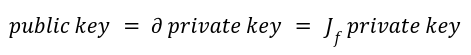Formula 1: Public key is the derivative of the private key. For the elliptic curve encryption algorithm the derivative is a Jacobian multiplication.

``````uint256 constant private GX = 0x79BE667EF9DCBBAC55A06295CE870B07029BFCDB2DCE28D959F2815B16F81798;
uint256 constant private GY = 0x483ADA7726A3C4655DA4FBFC0E1108A8FD17B448A68554199C47D08FFB10D4B8;
uint256 constant private AA = 0;
uint256 constant private PP = 0xFFFFFFFFFFFFFFFFFFFFFFFFFFFFFFFFFFFFFFFFFFFFFFFFFFFFFFFEFFFFFC2F;

function getPublicKey(uint256 privateKey) public pure returns (uint256, uint256) {
return getDeriveKey(privateKey, GX, GY);
}

function getDeriveKey(uint256 privateKey, uint256 gX, uint256 gY) public pure returns (uint256, uint256) {
return EllipticCurve.ecMul(
privateKey,
gX,
gY,
AA,
PP
);
}
``````

## Encryption with a private key on the blockchain by a smart contract.

The key point in this exchange is to validate that the given encrypted key could be a scam. We need to invalidate the sent key in the blockchain, for that, we need to perform encryption in the blockchain. The asymmetric encryption of a message is actually a symmetric encryption with a key that both the receiver and the sender can generate with the private and public keys of each other.

The key can be generated either by multiplying the sender's private key with the receiver's public key or by multiplying the receiver's private key with the sender's public key (see formula 2).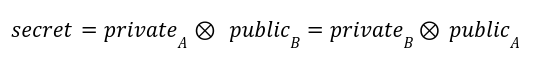Formula 2: Secret can be calculated by A and B with their respective private key by knowing the public key of the other.

The symmetric encryption is performed by the keccak256 hash operation. This operation is by default already implemented in the Solidity Language and therefore is cost-effective. You can see the method symmetricEncryption method below.

``````string constant COUNTER_VALUE = "7";

function symmetricEncryption(uint256 message, uint256 key) public pure returns (uint256) {
uint256 hash = uint256(keccak256(abi.encodePacked(key, COUNTER_VALUE)));
return message ^ hash;
}``````

Finally we can write our method to verify that the private key was invalid:

``````function dismiss(uint256 privateBuyerKey) public onlyBuyer onlyActive withResponse {
// Check that the given private buyer key is the true one.
(uint256 rbX, uint256 rbY) = Encrypt.getPublicKey(privateBuyerKey);
(uint256 bX, uint256 bY) = Encrypt.toUint256(buyerPublicKey);
require((rbX == bX) && (rbY == bY));
// Decrypt message to check the sent private article key.
uint256 wrongArticleKey = Encrypt.symmetricEncryption(Response(response).getEncryptedPrivateKey(), secretKey);
(uint256 waX, uint256 waY) = Encrypt.getPublicKey(wrongArticleKey);
(uint256 aX, uint256 aY) = Encrypt.toUint256(Article(article).getPublicKey());
require((waX != aX) || (waY != aY));
// Refund money and reject.
status = Status.REJECTED;
}
``````

``````function getSecret(uint256 privateKeyA, bytes memory publicKeyB) public pure returns (uint256) {
(uint256 gX, uint256 gY) = toUint256(publicKeyB);
(uint256 pX, ) = getDeriveKey(privateKeyA, gX, gY);
return pX;
}

function verifySignature(uint256 message, bytes memory signature, bytes memory pubKey) public pure returns (bool) {
return getSignatureTrace(message, signature) == getPubKeyTrace(pubKey);
}

function getSignatureTrace(uint256 message, bytes memory signature) public pure returns (address) {
return ECDSA.recover(bytes32(toBytes(message)), signature);
}

function getPubKeyTrace(bytes memory pubKey) public pure returns (address trace) {
bytes32 hash = keccak256(pubKey);
assembly { mstore(0, hash)  trace := mload(0) }
}

function bytesToBytes32(bytes memory b, uint offset) private pure returns (bytes32) {
bytes32 out;
for (uint i = 0; i < 32; i++) {
out |= bytes32(b[offset + i] & 0xFF) >> (i * 8);
}
return out;
}

function toBytes(uint256 x) private pure returns (bytes memory b) {
b = new bytes(32);
assembly { mstore(add(b, 32), x) }
}

function toUint256(bytes memory publicKeyB) public pure returns (uint256, uint256) {
uint256 x = uint256(bytesToBytes32(publicKeyB, 0));
uint256 y = uint256(bytesToBytes32(publicKeyB, 32));
return (x, y);
}
``````

This is the key method that allow the system to be secure and reliable.

Full code of the market place example can be found in this GitHub repository.

Sources